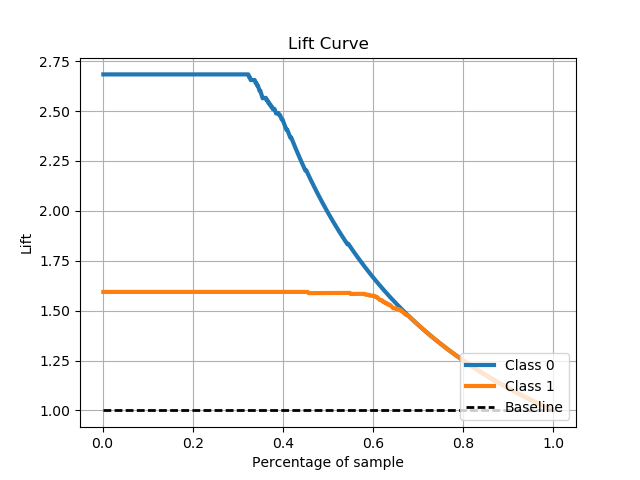# Metrics Module (API Reference)¶

The `scikitplot.metrics` module includes plots for machine learning evaluation metrics e.g. confusion matrix, silhouette scores, etc.

`scikitplot.metrics.``plot_confusion_matrix`(y_true, y_pred, labels=None, true_labels=None, pred_labels=None, title=None, normalize=False, hide_zeros=False, hide_counts=False, x_tick_rotation=0, ax=None, figsize=None, cmap='Blues', title_fontsize='large', text_fontsize='medium')

Generates confusion matrix plot from predictions and true labels

Parameters: y_true (array-like, shape (n_samples)) – Ground truth (correct) target values. y_pred (array-like, shape (n_samples)) – Estimated targets as returned by a classifier. labels (array-like, shape (n_classes), optional) – List of labels to index the matrix. This may be used to reorder or select a subset of labels. If none is given, those that appear at least once in `y_true` or `y_pred` are used in sorted order. (new in v0.2.5) true_labels (array-like, optional) – The true labels to display. If none is given, then all of the labels are used. pred_labels (array-like, optional) – The predicted labels to display. If none is given, then all of the labels are used. title (string, optional) – Title of the generated plot. Defaults to “Confusion Matrix” if normalize is True. Else, defaults to “Normalized Confusion Matrix. normalize (bool, optional) – If True, normalizes the confusion matrix before plotting. Defaults to False. hide_zeros (bool, optional) – If True, does not plot cells containing a value of zero. Defaults to False. hide_counts (bool, optional) – If True, doe not overlay counts. Defaults to False. x_tick_rotation (int, optional) – Rotates x-axis tick labels by the specified angle. This is useful in cases where there are numerous categories and the labels overlap each other. ax (`matplotlib.axes.Axes`, optional) – The axes upon which to plot the curve. If None, the plot is drawn on a new set of axes. figsize (2-tuple, optional) – Tuple denoting figure size of the plot e.g. (6, 6). Defaults to `None`. cmap (string or `matplotlib.colors.Colormap` instance, optional) – Colormap used for plotting the projection. View Matplotlib Colormap documentation for available options. https://matplotlib.org/users/colormaps.html title_fontsize (string or int, optional) – Matplotlib-style fontsizes. Use e.g. “small”, “medium”, “large” or integer-values. Defaults to “large”. text_fontsize (string or int, optional) – Matplotlib-style fontsizes. Use e.g. “small”, “medium”, “large” or integer-values. Defaults to “medium”. The axes on which the plot was drawn. ax (`matplotlib.axes.Axes`)

Example

```>>> import scikitplot as skplt
>>> rf = RandomForestClassifier()
>>> rf = rf.fit(X_train, y_train)
>>> y_pred = rf.predict(X_test)
>>> skplt.metrics.plot_confusion_matrix(y_test, y_pred, normalize=True)
<matplotlib.axes._subplots.AxesSubplot object at 0x7fe967d64490>
>>> plt.show()
```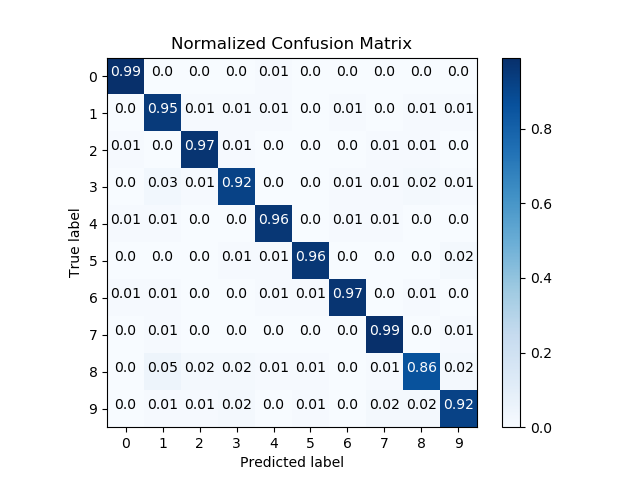`scikitplot.metrics.``plot_roc`(y_true, y_probas, title='ROC Curves', plot_micro=True, plot_macro=True, classes_to_plot=None, ax=None, figsize=None, cmap='nipy_spectral', title_fontsize='large', text_fontsize='medium')

Generates the ROC curves from labels and predicted scores/probabilities

Parameters: y_true (array-like, shape (n_samples)) – Ground truth (correct) target values. y_probas (array-like, shape (n_samples, n_classes)) – Prediction probabilities for each class returned by a classifier. title (string, optional) – Title of the generated plot. Defaults to “ROC Curves”. plot_micro (boolean, optional) – Plot the micro average ROC curve. Defaults to `True`. plot_macro (boolean, optional) – Plot the macro average ROC curve. Defaults to `True`. classes_to_plot (list-like, optional) – Classes for which the ROC curve should be plotted. e.g. [0, ‘cold’]. If given class does not exist, it will be ignored. If `None`, all classes will be plotted. Defaults to `None` ax (`matplotlib.axes.Axes`, optional) – The axes upon which to plot the curve. If None, the plot is drawn on a new set of axes. figsize (2-tuple, optional) – Tuple denoting figure size of the plot e.g. (6, 6). Defaults to `None`. cmap (string or `matplotlib.colors.Colormap` instance, optional) – Colormap used for plotting the projection. View Matplotlib Colormap documentation for available options. https://matplotlib.org/users/colormaps.html title_fontsize (string or int, optional) – Matplotlib-style fontsizes. Use e.g. “small”, “medium”, “large” or integer-values. Defaults to “large”. text_fontsize (string or int, optional) – Matplotlib-style fontsizes. Use e.g. “small”, “medium”, “large” or integer-values. Defaults to “medium”. The axes on which the plot was drawn. ax (`matplotlib.axes.Axes`)

Example

```>>> import scikitplot as skplt
>>> nb = GaussianNB()
>>> nb = nb.fit(X_train, y_train)
>>> y_probas = nb.predict_proba(X_test)
>>> skplt.metrics.plot_roc(y_test, y_probas)
<matplotlib.axes._subplots.AxesSubplot object at 0x7fe967d64490>
>>> plt.show()
```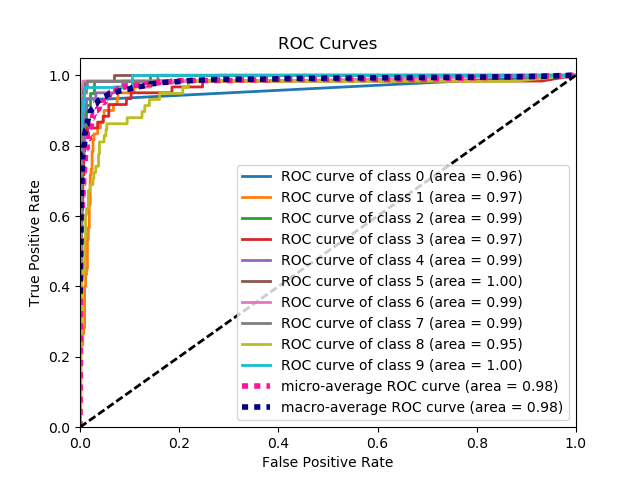`scikitplot.metrics.``plot_ks_statistic`(y_true, y_probas, title='KS Statistic Plot', ax=None, figsize=None, title_fontsize='large', text_fontsize='medium')

Generates the KS Statistic plot from labels and scores/probabilities

Parameters: y_true (array-like, shape (n_samples)) – Ground truth (correct) target values. y_probas (array-like, shape (n_samples, n_classes)) – Prediction probabilities for each class returned by a classifier. title (string, optional) – Title of the generated plot. Defaults to “KS Statistic Plot”. ax (`matplotlib.axes.Axes`, optional) – The axes upon which to plot the learning curve. If None, the plot is drawn on a new set of axes. figsize (2-tuple, optional) – Tuple denoting figure size of the plot e.g. (6, 6). Defaults to `None`. title_fontsize (string or int, optional) – Matplotlib-style fontsizes. Use e.g. “small”, “medium”, “large” or integer-values. Defaults to “large”. text_fontsize (string or int, optional) – Matplotlib-style fontsizes. Use e.g. “small”, “medium”, “large” or integer-values. Defaults to “medium”. The axes on which the plot was drawn. ax (`matplotlib.axes.Axes`)

Example

```>>> import scikitplot as skplt
>>> lr = LogisticRegression()
>>> lr = lr.fit(X_train, y_train)
>>> y_probas = lr.predict_proba(X_test)
>>> skplt.metrics.plot_ks_statistic(y_test, y_probas)
<matplotlib.axes._subplots.AxesSubplot object at 0x7fe967d64490>
>>> plt.show()
```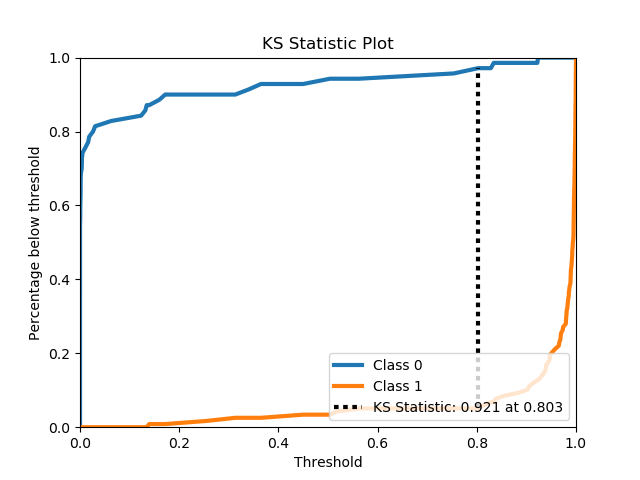`scikitplot.metrics.``plot_precision_recall`(y_true, y_probas, title='Precision-Recall Curve', plot_micro=True, classes_to_plot=None, ax=None, figsize=None, cmap='nipy_spectral', title_fontsize='large', text_fontsize='medium')

Generates the Precision Recall Curve from labels and probabilities

Parameters: y_true (array-like, shape (n_samples)) – Ground truth (correct) target values. y_probas (array-like, shape (n_samples, n_classes)) – Prediction probabilities for each class returned by a classifier. title (string, optional) – Title of the generated plot. Defaults to “Precision-Recall curve”. plot_micro (boolean, optional) – Plot the micro average ROC curve. Defaults to `True`. classes_to_plot (list-like, optional) – Classes for which the precision-recall curve should be plotted. e.g. [0, ‘cold’]. If given class does not exist, it will be ignored. If `None`, all classes will be plotted. Defaults to `None`. ax (`matplotlib.axes.Axes`, optional) – The axes upon which to plot the curve. If None, the plot is drawn on a new set of axes. figsize (2-tuple, optional) – Tuple denoting figure size of the plot e.g. (6, 6). Defaults to `None`. cmap (string or `matplotlib.colors.Colormap` instance, optional) – Colormap used for plotting the projection. View Matplotlib Colormap documentation for available options. https://matplotlib.org/users/colormaps.html title_fontsize (string or int, optional) – Matplotlib-style fontsizes. Use e.g. “small”, “medium”, “large” or integer-values. Defaults to “large”. text_fontsize (string or int, optional) – Matplotlib-style fontsizes. Use e.g. “small”, “medium”, “large” or integer-values. Defaults to “medium”. The axes on which the plot was drawn. ax (`matplotlib.axes.Axes`)

Example

```>>> import scikitplot as skplt
>>> nb = GaussianNB()
>>> nb.fit(X_train, y_train)
>>> y_probas = nb.predict_proba(X_test)
>>> skplt.metrics.plot_precision_recall(y_test, y_probas)
<matplotlib.axes._subplots.AxesSubplot object at 0x7fe967d64490>
>>> plt.show()
```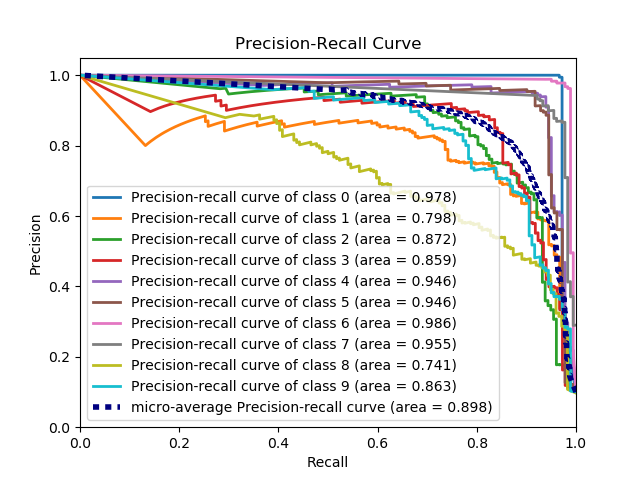`scikitplot.metrics.``plot_silhouette`(X, cluster_labels, title='Silhouette Analysis', metric='euclidean', copy=True, ax=None, figsize=None, cmap='nipy_spectral', title_fontsize='large', text_fontsize='medium')

Plots silhouette analysis of clusters provided.

Parameters: X (array-like, shape (n_samples, n_features)) – Data to cluster, where n_samples is the number of samples and n_features is the number of features. cluster_labels (array-like, shape (n_samples,)) – Cluster label for each sample. title (string, optional) – Title of the generated plot. Defaults to “Silhouette Analysis” metric (string or callable, optional) – The metric to use when calculating distance between instances in a feature array. If metric is a string, it must be one of the options allowed by sklearn.metrics.pairwise.pairwise_distances. If X is the distance array itself, use “precomputed” as the metric. copy (boolean, optional) – Determines whether `fit` is used on clf or on a copy of clf. ax (`matplotlib.axes.Axes`, optional) – The axes upon which to plot the curve. If None, the plot is drawn on a new set of axes. figsize (2-tuple, optional) – Tuple denoting figure size of the plot e.g. (6, 6). Defaults to `None`. cmap (string or `matplotlib.colors.Colormap` instance, optional) – Colormap used for plotting the projection. View Matplotlib Colormap documentation for available options. https://matplotlib.org/users/colormaps.html title_fontsize (string or int, optional) – Matplotlib-style fontsizes. Use e.g. “small”, “medium”, “large” or integer-values. Defaults to “large”. text_fontsize (string or int, optional) – Matplotlib-style fontsizes. Use e.g. “small”, “medium”, “large” or integer-values. Defaults to “medium”. The axes on which the plot was drawn. ax (`matplotlib.axes.Axes`)

Example

```>>> import scikitplot as skplt
>>> kmeans = KMeans(n_clusters=4, random_state=1)
>>> cluster_labels = kmeans.fit_predict(X)
>>> skplt.metrics.plot_silhouette(X, cluster_labels)
<matplotlib.axes._subplots.AxesSubplot object at 0x7fe967d64490>
>>> plt.show()
```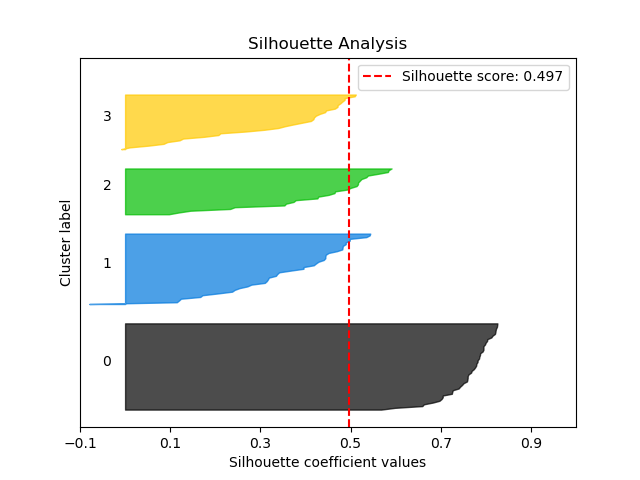`scikitplot.metrics.``plot_calibration_curve`(y_true, probas_list, clf_names=None, n_bins=10, title='Calibration plots (Reliability Curves)', ax=None, figsize=None, cmap='nipy_spectral', title_fontsize='large', text_fontsize='medium')

Plots calibration curves for a set of classifier probability estimates.

Plotting the calibration curves of a classifier is useful for determining whether or not you can interpret their predicted probabilities directly as as confidence level. For instance, a well-calibrated binary classifier should classify the samples such that for samples to which it gave a score of 0.8, around 80% should actually be from the positive class.

This function currently only works for binary classification.

Parameters: y_true (array-like, shape (n_samples)) – Ground truth (correct) target values. probas_list (list of array-like, shape (n_samples, 2) or (n_samples,)) – A list containing the outputs of binary classifiers’ `predict_proba()` method or `decision_function()` method. clf_names (list of str, optional) – A list of strings, where each string refers to the name of the classifier that produced the corresponding probability estimates in probas_list. If `None`, the names “Classifier 1”, “Classifier 2”, etc. will be used. n_bins (int, optional) – Number of bins. A bigger number requires more data. title (string, optional) – Title of the generated plot. Defaults to “Calibration plots (Reliabilirt Curves)” ax (`matplotlib.axes.Axes`, optional) – The axes upon which to plot the curve. If None, the plot is drawn on a new set of axes. figsize (2-tuple, optional) – Tuple denoting figure size of the plot e.g. (6, 6). Defaults to `None`. cmap (string or `matplotlib.colors.Colormap` instance, optional) – Colormap used for plotting the projection. View Matplotlib Colormap documentation for available options. https://matplotlib.org/users/colormaps.html title_fontsize (string or int, optional) – Matplotlib-style fontsizes. Use e.g. “small”, “medium”, “large” or integer-values. Defaults to “large”. text_fontsize (string or int, optional) – Matplotlib-style fontsizes. Use e.g. “small”, “medium”, “large” or integer-values. Defaults to “medium”. The axes on which the plot was drawn. `matplotlib.axes.Axes`

Example

```>>> import scikitplot as skplt
>>> rf = RandomForestClassifier()
>>> lr = LogisticRegression()
>>> nb = GaussianNB()
>>> svm = LinearSVC()
>>> rf_probas = rf.fit(X_train, y_train).predict_proba(X_test)
>>> lr_probas = lr.fit(X_train, y_train).predict_proba(X_test)
>>> nb_probas = nb.fit(X_train, y_train).predict_proba(X_test)
>>> svm_scores = svm.fit(X_train, y_train).decision_function(X_test)
>>> probas_list = [rf_probas, lr_probas, nb_probas, svm_scores]
>>> clf_names = ['Random Forest', 'Logistic Regression',
...              'Gaussian Naive Bayes', 'Support Vector Machine']
>>> skplt.metrics.plot_calibration_curve(y_test,
...                                      probas_list,
...                                      clf_names)
<matplotlib.axes._subplots.AxesSubplot object at 0x7fe967d64490>
>>> plt.show()
```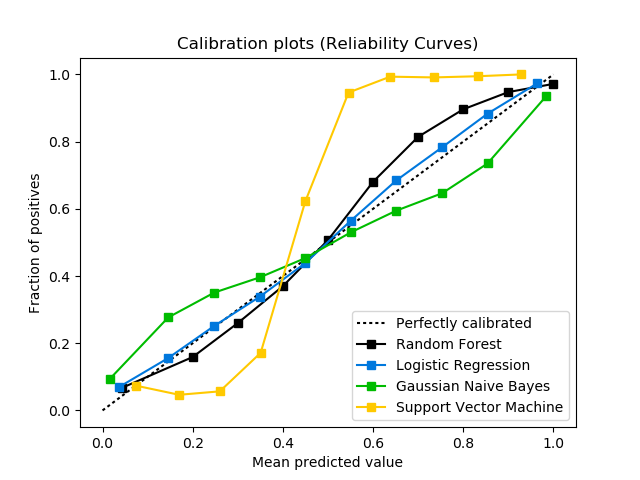`scikitplot.metrics.``plot_cumulative_gain`(y_true, y_probas, title='Cumulative Gains Curve', ax=None, figsize=None, title_fontsize='large', text_fontsize='medium')

Generates the Cumulative Gains Plot from labels and scores/probabilities

The cumulative gains chart is used to determine the effectiveness of a binary classifier. A detailed explanation can be found at http://mlwiki.org/index.php/Cumulative_Gain_Chart. The implementation here works only for binary classification.

Parameters: y_true (array-like, shape (n_samples)) – Ground truth (correct) target values. y_probas (array-like, shape (n_samples, n_classes)) – Prediction probabilities for each class returned by a classifier. title (string, optional) – Title of the generated plot. Defaults to “Cumulative Gains Curve”. ax (`matplotlib.axes.Axes`, optional) – The axes upon which to plot the learning curve. If None, the plot is drawn on a new set of axes. figsize (2-tuple, optional) – Tuple denoting figure size of the plot e.g. (6, 6). Defaults to `None`. title_fontsize (string or int, optional) – Matplotlib-style fontsizes. Use e.g. “small”, “medium”, “large” or integer-values. Defaults to “large”. text_fontsize (string or int, optional) – Matplotlib-style fontsizes. Use e.g. “small”, “medium”, “large” or integer-values. Defaults to “medium”. The axes on which the plot was drawn. ax (`matplotlib.axes.Axes`)

Example

```>>> import scikitplot as skplt
>>> lr = LogisticRegression()
>>> lr = lr.fit(X_train, y_train)
>>> y_probas = lr.predict_proba(X_test)
>>> skplt.metrics.plot_cumulative_gain(y_test, y_probas)
<matplotlib.axes._subplots.AxesSubplot object at 0x7fe967d64490>
>>> plt.show()
```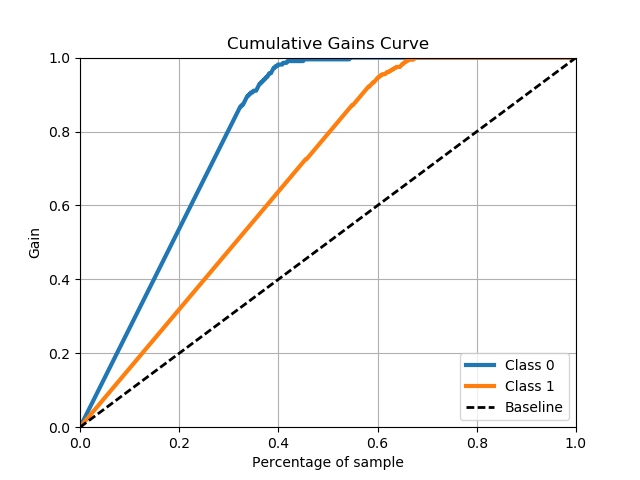`scikitplot.metrics.``plot_lift_curve`(y_true, y_probas, title='Lift Curve', ax=None, figsize=None, title_fontsize='large', text_fontsize='medium')

Generates the Lift Curve from labels and scores/probabilities

The lift curve is used to determine the effectiveness of a binary classifier. A detailed explanation can be found at http://www2.cs.uregina.ca/~dbd/cs831/notes/lift_chart/lift_chart.html. The implementation here works only for binary classification.

Parameters: y_true (array-like, shape (n_samples)) – Ground truth (correct) target values. y_probas (array-like, shape (n_samples, n_classes)) – Prediction probabilities for each class returned by a classifier. title (string, optional) – Title of the generated plot. Defaults to “Lift Curve”. ax (`matplotlib.axes.Axes`, optional) – The axes upon which to plot the learning curve. If None, the plot is drawn on a new set of axes. figsize (2-tuple, optional) – Tuple denoting figure size of the plot e.g. (6, 6). Defaults to `None`. title_fontsize (string or int, optional) – Matplotlib-style fontsizes. Use e.g. “small”, “medium”, “large” or integer-values. Defaults to “large”. text_fontsize (string or int, optional) – Matplotlib-style fontsizes. Use e.g. “small”, “medium”, “large” or integer-values. Defaults to “medium”. The axes on which the plot was drawn. ax (`matplotlib.axes.Axes`)

Example

```>>> import scikitplot as skplt
>>> lr = LogisticRegression()
>>> lr = lr.fit(X_train, y_train)
>>> y_probas = lr.predict_proba(X_test)
>>> skplt.metrics.plot_lift_curve(y_test, y_probas)
<matplotlib.axes._subplots.AxesSubplot object at 0x7fe967d64490>
>>> plt.show()
```